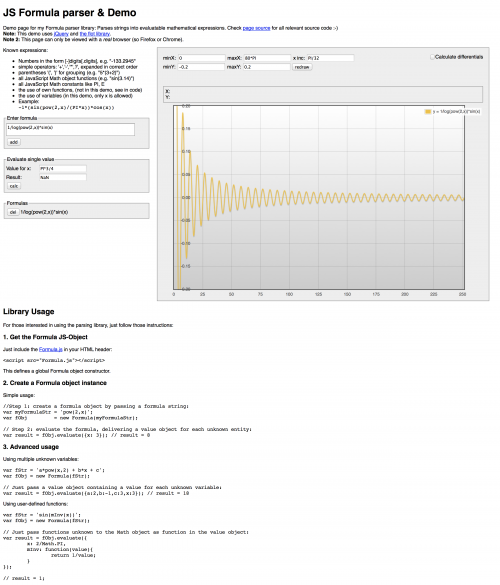# JS Formula Parser

Check me out on github

A formula parser written in JavaScript. It takes a string, and tries to parse the mathematical formula within, and generates a machine traversable resolving tree, respectively lets you easily evaluate the parsed formulas with numbers.

## Some features

• knows integers, floats
• simple operators like (+, -, *, /) parsed in the correct order
• parentheses for grouping (e.g. 5*(3+8))
• all JavaScript Math functions and constants (like sin, PI)
• definition of own functions and variables

Check out the parser in action here: